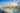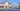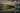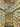# Visualising Decoding Algorithms

#### Desktop Version | 21 July, 2019

In the neural image caption generator post, we saw how to build and train the neural network that can generate the caption for any given image. We also saw how the choice of decoder impacts the quality of captions generated. And whilst we described how each decoder works, in words, I find it easier to understand the concepts when they are visualized.

Recollect that after we have trained our image caption model, we initiate the caption generation process by feeding the image tensor along with the special start of sentence token (i.e. <START>). The model generates the probability distribution (actually the logits) over our vocabulary of 36,780 words. The orange box shows the choice of decoding algorithms that helps us choose which word to use. The chosen word and the image is then passed again to the model until we meet the stopping criteria which are either we get the special end of sentence token (i.e. <STOP>) as our next word or we exceed the predefined number of steps where one step is passing the image and word tensor to the caption generator model and choosing the word using decoding algorithm.

In this post, we focus on the orange box i.e the decoding algorithms that help us choose the word from a probability distribution over the entire vocabulary.

## Greedy Decoder

This is the most straightforward approach where we select the word that has the highest probability (i.e act greedily). And while it could generate the sequence of words, the quality of output is often low when compared to the other decoding algorithms. You can check out the "Test Drive" section under the image caption post for comparison.Silo Park, Auckland: Input image to test Greedy Decoder

Greedy decoder in action: argmax)

It is not possible to show all 36,780 words from the vocabulary, so we pick the top 60 words for the visualization purpose. Also, note that it causes the labels to switch at every timestep but hopefully still conveys the idea.

## Beam Search Decoder

In the greedy decoder, we considered a single word at every step. What if we could track multiple words at every step and use those to generate multiple hypotheses.

This is exactly what the beam search algorithm does, we define how many words (k) we want to keep at every step. The algorithm keeps track of k words along with its score, each seeded from the previous top scoring k words. The score is calculated as a sum of the log probability of the hypothesis generated so far.

$score(y_1,...,y_t)= \sum_{i=1}^t logP(y_i|y_1,....,y_{i-1}, x)$

where t is the step, x is the input image and y are the words generated. The stopping criteria remain the same as greedy search where the hypothesis stops as soon as we encounter <STOP> or run out of a predefined maximum number of steps.The end result is a tree of words (i.e multiple hypotheses), and we pick the one that has the highest score as our final solution.

When we use k=1, it works just like the greedy decoder algorithm and suffers from the same issue of producing low-quality output. As we increase k the algorithm starts to generate better quality output, although at larger k the output gets very short. Also, note that increasing k is compute-intensive because we need to keep track of k words at every step.Queenstown: Input image to test beam search decoder

Beam search decoder with k=3 and max steps as 51

The start and stop words are highlighted in green and red, and the grey text shows the score of sequence at that step or point in time.

You may find this post on effect of parameters on beam search useful.

## Pure Sampling Decoder

Pure sampling decoder is very similar to the greedy search decoder, but instead of picking the word with the highest probability, we randomly sample the word from the probability distribution of entire vocabulary. The sampling methods such as pure sampling and Top-K sampling (below) provide better diversity and are generally considered better at generating natural language.Marina Bay, Singapore: Input image to test pure sampling decoder

Pure sampling decoder: orange - selected word and blue - highest probability word

## Top-K Sampling Decoder

This approach is similar to the Pure sampling decoder, but instead of using the entire probability distribution, we use top-k probable words. If we use k=1, it is same as greedy search and if we use the total length of vocabulary as k then it works as pure sampling decoder. The visualization below uses the same input image as the pure sampling example.

Top-K sampling decoder with k=8, orange - selected word and blue - top-k words with highest probability

Conclusion

And that concludes the visualization of various decoding algorithms I used in my post  on neural image caption generation. Here is one last example showing the output of all four decoders for the same input image.Greedy
BEAM SEARCH
PURE
TOP-K
References:
1. My Post on Neural Image Caption Generator  [link]
2. Show and Tell: A Neural Image Caption Generator  [arvix]
3. CS224N Machine Translation Lecture  [link]